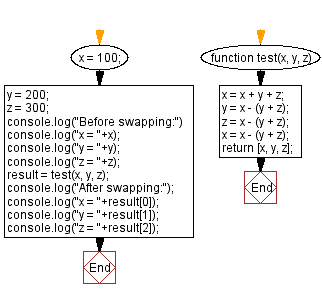# JavaScript: Swap three numbers

## JavaScript Math: Exercise-75 with Solution

Write a JavaScript program to swap three numbers without a third variable.

Test Data:
(100, 200, 300) -> 300, 100, 200

Sample Solution:

HTML Code:

``````<!DOCTYPE html>
<html>
<meta charset="utf-8">
<title>JavaScript program to Swap three numbers</title>
<body>

</body>
</html>
```
```

JavaScript Code:

``````function test(x, y, z)
{
x = x + y + z;
y = x - (y + z);
z = x - (y + z);
x = x - (y + z);
return [x, y, z];
}

x = 100;
y = 200;
z = 300;
console.log("Before swapping:")
console.log("x = "+x);
console.log("y = "+y);
console.log("z = "+z);
result = test(x, y, z);
console.log("After swapping:");
console.log("x = "+result);
console.log("y = "+result);
console.log("z = "+result);
```
```

Sample Output:

```Before swapping:
x = 100
y = 200
z = 300
After swapping:
x = 300
y = 100
z = 200
```

Flowchart:Live Demo:

See the Pen javascript-math-exercise-75 by w3resource (@w3resource) on CodePen.

Improve this sample solution and post your code through Disqus

What is the difficulty level of this exercise?

Test your Programming skills with w3resource's quiz.

﻿